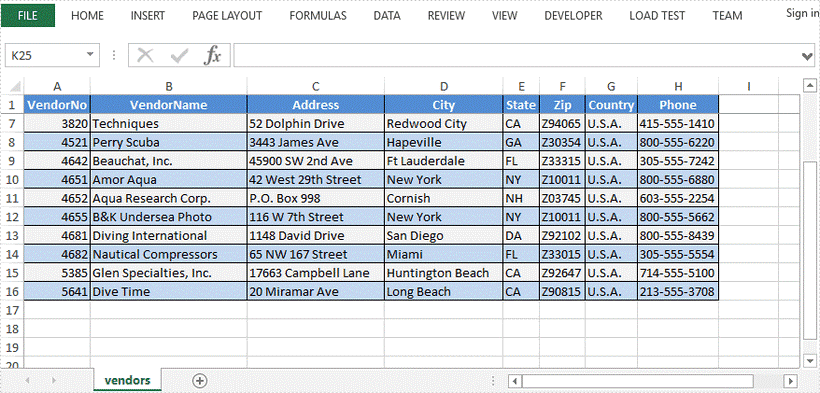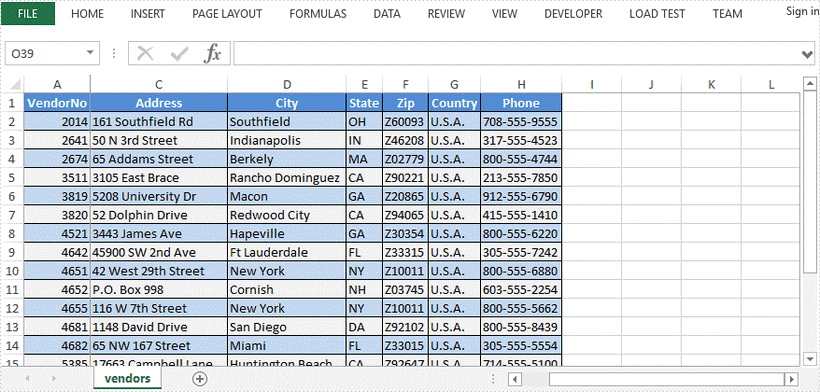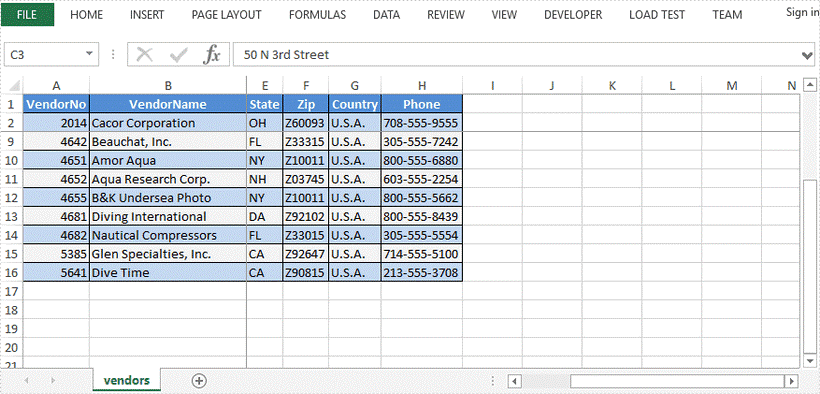# Freeze or Unfreeze Excel Rows and Columns in Java

This article demonstrates how to freeze or unfreeze rows and columns in Excel using Spire.XLS for Java.

Freeze top row

```//Create a Workbook instance
Workbook workbook = new Workbook();

//Get the first worksheet
Worksheet sheet = workbook.getWorksheets().get(0);

//Freeze top row
sheet.freezePanes(2,1);

//Save to file
workbook.saveToFile("FreezeTopRow.xlsx", ExcelVersion.Version2016);
```Freeze fisrt column

```//Create a Workbook instance
Workbook workbook = new Workbook();

//Get the first worksheet
Worksheet sheet = workbook.getWorksheets().get(0);

//Freeze frist column
sheet.freezePanes(1,2);

//Save to file
workbook.saveToFile("FreezeFirstColumn.xlsx", ExcelVersion.Version2016);
```Freeze few rows and columns

```//Create a Workbook instance
Workbook workbook = new Workbook();

//Get the first worksheet
Worksheet sheet = workbook.getWorksheets().get(0);

//Freeze the second row and the second column
sheet.freezePanes(3,3);

//Save to file
workbook.saveToFile("FreezeFewRowsAndColumns.xlsx", ExcelVersion.Version2016);
```Unfreeze panes

```//Create a Workbook instance
Workbook workbook = new Workbook();